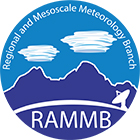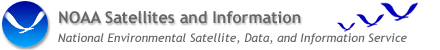## GOES-R Application Development

### Probabilistic Assimilation and Prediction Framework - MLEF

An ensemble based data assimilation and prediction framework has been developed at Colorado State University. This fully probabilistic approach to data assimilation and prediction, based on estimation theory, is formulated as Maximum Likelihood Ensemble Filter (Zupanski 2005; Zupanski and Zupanski 2005) and denoted MLEF framework. The MLEF framework provides maximum likelihood solution to the atmospheric state, model errors and empirical parameters, employing an iterative minimization. It also calculates the analysis and forecast error covariance matrices as measures of analysis and forecast uncertainty. In application to GOES-R and NPP risk reduction programs, the Regional Atmospheric Modeling System (RAMS) has been included into the MLEF framework. The probabilistic MLEF framework will be used to determine the analysis and forecast uncertainty, as produced by RAMS model, employing simulated GOES-R observations. One of the goals of this project is to measure value added of the GOES-R observations in terms of information content of these new observations.

### PCI Analysis

Basics of Principal Component Image transformation

Principal Components (PCs) have the ability to simplify multivariate data by reducing the dimensionality of the data set (Gauch 1993). Features that are hidden in the data are brought out by PC analysis (Loughlin 1991). PC theory dictates that the information content of the PCs is compressed into the PCs in order of descending significance, with the lower-numbered PCs containing the primary information content, and the higher-numbered PCs containing other information and noise. Both Morrison (1976) and Preisendorfer (1988) give good graphical representations of the PC transformation process. The process can be summarized as a translation and rotation of the original coordinate system into a new coordinate system that better reflects the principal modes of variability in the data set being analyzed.

Because of its ability to simplify multi-spectral data sets, PC (or eigenvector/eigenvalue analysis) analysis has been used extensively for the analysis of high-spatial-resolution environmental (land and ocean) remote-sensing imagery. However, the technique can also be used to analyze the information content of lower-spatial-resolution weather satellite imagery and has been termed Principal Component Images (PCIs) by Hillger (1996). Regardless of the intended application, the technique determines which part of the multi spectral signal is common to all the images (spectral bands) and separates that information from other image information that is sensed only by image differences or multiple image combinations. Whereas the original images may (and often do) contain redundant information, the PCIs contain the independent signal separated out of the original images. This allows the image analyst to see the independent components of multi spectral imagery.

Application to Satellite Imagery

The process of transforming multi-band satellite imagery into PCIs is based on statistics generated from the images. Consider a set of imagery from N bands, viewing a scene at M horizontal locations (pixels, which includes data collected in scan lines and with a large number of samples in each line). At each pixel or location a vector of length N, denoted by B, can represent the multi-band imagery. A special linear transformation can be applied to provide a new vector of length N, denoted PCI as follows

PCI = E · B

where E is an N by N matrix. For PCIs, the rows of E are the eigenvectors of the symmetric N x N covariance matrix with elements composed of covariances among the bands (summed over M pixel locations). The covariance matrix is generated from the imagery (or a subset of the imagery) being analyzed, and the eigenvectors are determined using a standard mathematical package for diagonalizing that matrix. The covariance matrix explains the relationships among the band images, allowing the eigenvector transform to parse that information into the PCIs. This parsing separates common and difference information from the multi-spectral imagery. The common information is concentrated into the PCIs in order of decreasing explained variance (the square of the eigenvalue), with PCI-1 containing most of the variance, and lesser variance in higher-numbered PCIs. The result of the eigenvector transformation is a restructuring of the satellite information into as many PCIs as there are available spectral-band images. (The PCIs can have no more degrees of freedom than the bands images that are input.) The sum of the explained variances of the PCIs is equal to the sum of the explained variances of the original images, the same information content as the original imagery expressed in a new form.

The PCI concept is easier to explain when simplified to a small number of images or dimensions. In the simplest two dimensional case, two band images, b1 and b2, are transformed into two PCIs, pci1 and pci2, using

pci1 = e1 · b1 + e2 · b2

and

pci2 = f1 · b1 + f2 · b2

where e and f are linear transformation vectors (eigenvectors, or rows in the eigenvector matrix E) used to transform each pixel (or picture element) in the original band images into two PCIs. The individual e's and f's (eigenvector coefficients) can be positive or negative, for adding or subtracting the bands, as required by the transformation from bands into PCIs. With only two input bands, pci1 contains the information that is common to the b1 and b2 images (an image sum), and pci2 contains the information that is not shared, or that differs between, the b1 and b2 images (an image difference). The three band case can be visualized as a transformation of axes in three dimensional space. For increasing numbers of images the transformation is increasingly harder to visualize.

An Example of 2-band PCI Analysis

Examples of PCI analysis of satellite images for the simplest two-band case are given in Figures 1 and 2. Figure 1a,b contains two infrared window band images (from MODIS) at 3.9 and 11.0 mm (a shortwave infrared and a longwave infrared band) respectively. These two window band images generally show similar features, but with differences between the two bands due to variations in the emittance of the land and cloud features.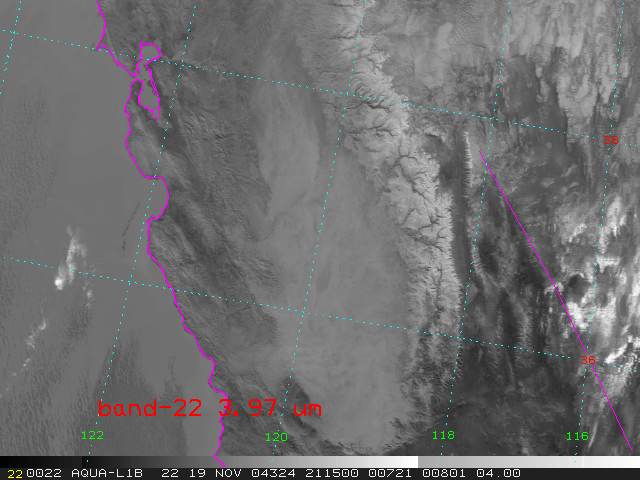Figure 1a. MODIS shortwave infrared Band 22 (3.97 mm) showing fog in the central valley of California. Click the image for a larger version.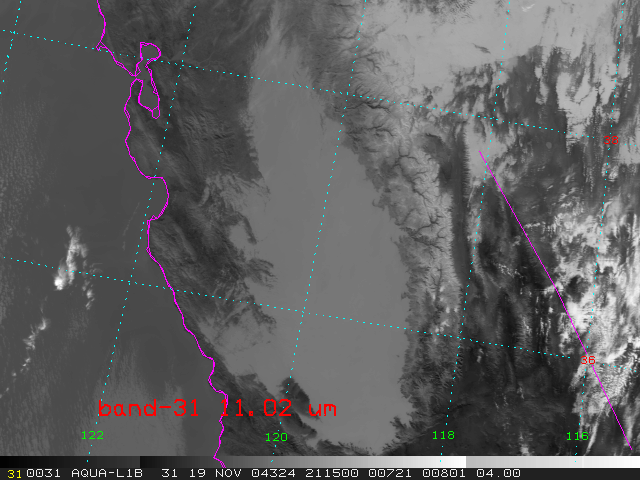Figure 1b. MODIS infrared Band 31 (11.02 mm). Click the image for a larger version.

The two PCIs generated from the two images in Figure 1a,b are shown in Figure 2a,b. PCI-1 is a weighted-sum (or weighted-average) of the two band images. Being a weighted-sum, it looks similar to both of the input bands, extracting features common to the two window band images. PCI-2 is a weighted-difference image, highlighting where the two window band images are the most different. This PCI is similar to other image products that difference the two infrared window bands, such as either the fog/reflectivity product or the shortwave albedo product.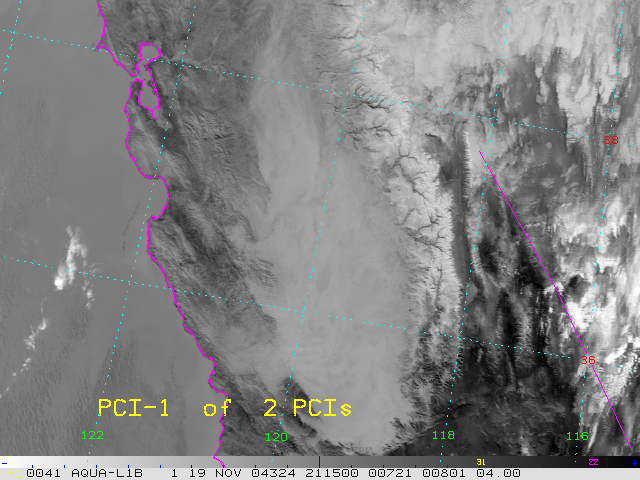Figure 2a. PCI-1 generated from the two bands shown in Fig. 1a,b. Click the image for a larger version.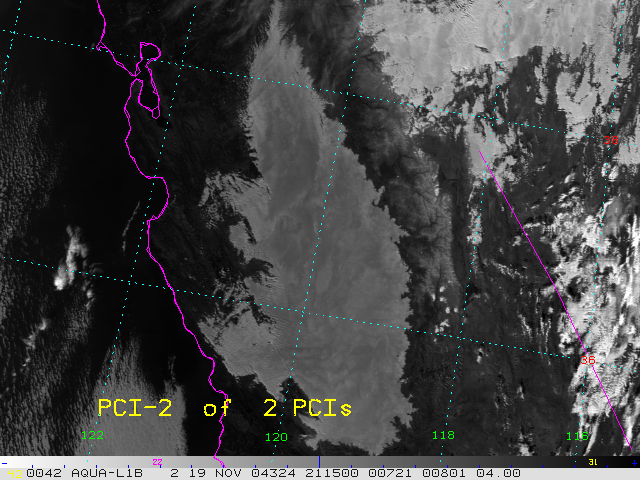Figure 2b. PCI-2 generated from the two bands shown in Fig. 1a,b. Click the image for a larger version.

An Example of 3-band PCI Analysis

Examples of PCI analysis of satellite images for a three-band case are given in Figures 3 and 4. Figure 3a,b,c contains three window-band images (from MODIS) at 0.6, 3.9, and 11.0 mm (a visible, a shortwave infrared, and a longwave infrared band) respectively. These three window-band images generally show similar features, but with differences among the three bands due to variations in the emittance and reflectance of the land and cloud features.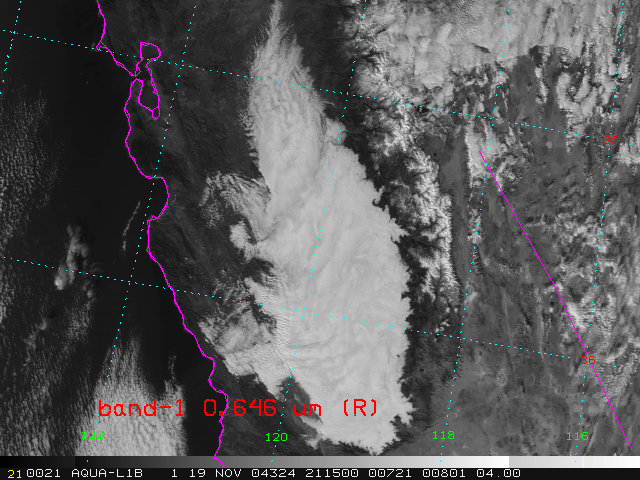Figure 3a. MODIS visible band 1 (0.646 mm). Click the image for a larger version.Figure 3b. MODIS shortwave infrared Band 22 (3.97 mm). Click the image for a larger version.Figure 3c. MODIS infrared Band 31 (11.02 mm). Click the image for a larger version.

The three PCIs generated from the three images in Figure 3 are shown in Figure 4a,b,c. PCI-1 is a weighted-sum (or weighted-average) separating the information that is common to, or redundant among, the three band images, and explaining most of the variance in the three input bands. Being a weighted-sum, it looks similar to the input bands, extracting features common to the three window-band images. PCI-2 is a weighted-sum-and-difference image, highlighting where the three window-band images differ the most, and explaining the majority of the remaining variance among the three input bands. PCI-3 is a final weighted-sum-and-difference image, with the remainder of the explained variance among the three input bands. These three PCIs contain the same information as the three input bands, but rearranged by separating the common and difference information.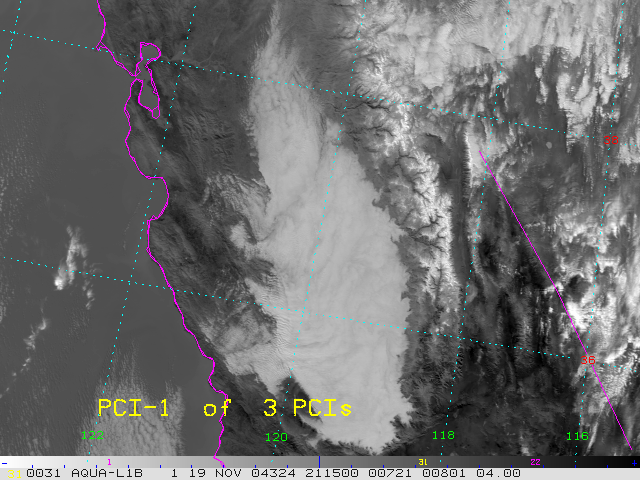Figure 4a. PCI-1 generated from the three bands shown in Fig. 3a,b,c. Click the image for a larger version.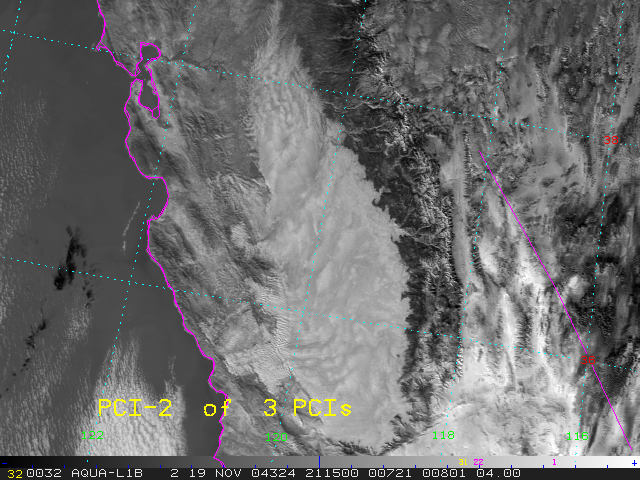Figure 4b. PCI-2 generated from the three bands shown in Fig. 3a,b,c. Click the image for a larger version.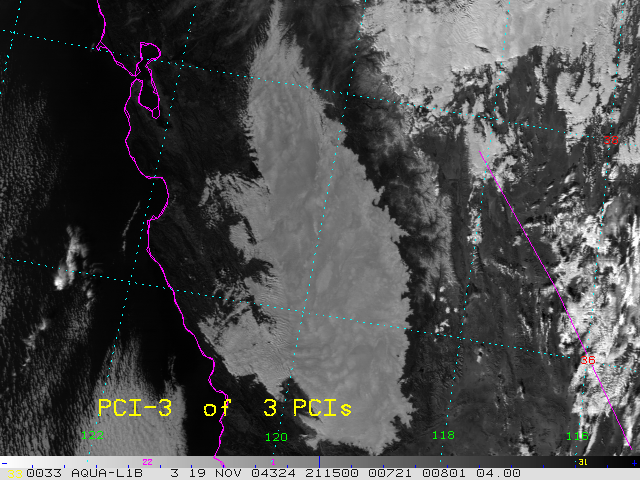Figure 4c. PCI-3 generated from the three bands shown in Fig. 3a,b,c. Click the image for a larger version.

Color Combinations of bands and PCIs

Three-color combinations of multi-band imagery are sometimes used to help separate features in the images that are spectrally different. Figure 5a is an example of a three-color combination of the three window-band images in Figure 3. The colors help separate the fog from the land and the snow-covered mountains. Figure 5b is a similar three-color combination of the three PCIs in Figure 4. This three-color combination seems to better separate the different surface types, due to the fact that the PCIs have already reduced the redundancy among the three input band images, and separated the major blocks of explained variance. The fog is now more clearly separated from the land and the snow-covered mountains, and there is more detail in the features that do appear. This shows the advantage of doing PCI analysis first.Figure 5a. Three-color combination of the three window-band images in Figure 3. Click the image for a larger version.Figure 5b. Thee-color combination of the three PCIs in Figure 4. Click the image for a larger version.

### References

Gauch, H.G. Jr., 1993: Prediction, parsimony, and noise. American Scientist, 81(5), 468-478.

Hillger, D.W., 1996: Meteorological features from principal component image transformation of GOES imagery. International Symposium on Optical Science, Engineering, and Instrumentation (GOES-8 and Beyond Conference), SPIE, volume 2812, 4-9 August, Denver CO, 111-121.

Loughlin, W.P., 1991: Principal component analysis for alteration mapping. Photogrammetric Engineering and Remote Sensing, 57(9), 1163-1169.

Morrison, D.F., 1976: Multivariate Statistical Methods. McGraw-Hill, New York, 415 pp.

Preisendorfer, R.W., 1988: Principal Component Analysis in Meteorology and Oceanography. Elsevier, New York, 425 pp.